/NumPy 1.14

# numpy.random.chisquare

numpy.random.chisquare(df, size=None)

Draw samples from a chi-square distribution.

When df independent random variables, each with standard normal distributions (mean 0, variance 1), are squared and summed, the resulting distribution is chi-square (see Notes). This distribution is often used in hypothesis testing.

Parameters: df : float or array_like of floats Number of degrees of freedom, should be > 0. size : int or tuple of ints, optional Output shape. If the given shape is, e.g., (m, n, k), then m * n * k samples are drawn. If size is None (default), a single value is returned if df is a scalar. Otherwise, np.array(df).size samples are drawn. out : ndarray or scalar Drawn samples from the parameterized chi-square distribution. ValueError When df <= 0 or when an inappropriate size (e.g. size=-1) is given.

#### Notes

The variable obtained by summing the squares of df independent, standard normally distributed random variables: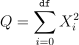is chi-square distributed, denoted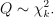The probability density function of the chi-squared distribution is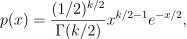where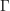is the gamma function,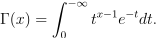#### References

 [R427427] NIST “Engineering Statistics Handbook” http://www.itl.nist.gov/div898/handbook/eda/section3/eda3666.htm

#### Examples

>>> np.random.chisquare(2,4)
array([ 1.89920014,  9.00867716,  3.13710533,  5.62318272])


© 2008–2017 NumPy Developers